# HC Verma Solutions Class 11 Chapter 22 Photometry

HC Verma Solutions Class 11 Chapter 22 Photometry provided here will enable students to enhance their problem-solving skills in tackling questions given in the chapter and question that are related to topics like radiant flux, relative luminosity and luminous flux of source, etc.

However, in this chapter students will be dealing with problems like;

• Questions related to finding the luminous efficiency when luminous flux and radiant flux are given and vice versa.
• Questions related to illuminance along with the luminous intensity of source where students will be finding the distance between the source and the original position.

In order to help students understand the concept clearly and solve physics problems with ease, we are offering free HC Verma Solutions for class 11 below. The solutions consist of answers to all the exercises found in chapter 22 of HC Verma book vol 1. Students can use the solutions to understand the topics more clearly and learn the right way to tackle questions. Moreover, students can refer the solutions to study well for the exams.

 What is Luminous Efficiency? The concept behind a light source able to generate visible light is termed as luminous efficiency. What is Radiant Flux? It is referred to the tendency of the energy that is radiated to emit, reflect, transmit, and receive with respect to per unit time.

2. The luminosity of Radiant Flux 449
3. Luminous Flux: Relative Luminosity 449
4. Luminous Efficiency
5. Luminous Intensity or Illuminating Power 450
6. Illuminance
7. Inverse Square Law 451
8. Lambert’s Cosine Law
9. Photometers

## Class 11 Important Questions In Chapter 22

1. What are your thoughts about the statement, “a 1 W sodium vapour lamp has a luminous flux of more than that of 10 kW source of ultraviolet radiation.” Give reasons.

2. Can you explain why the sun is less bright in the morning and evening as compared to at noon? Remember the distance of the sun from the observer is almost the same.

3. As the wavelength is increased from violet to red, the luminosity (a) continuously increases (b) continuously decreases (c) increases then decreases (d) decreases then increases.

4. A photographic plate is kept at 5 cm apart from a weak point source and is then exposed for 3 s. What will be the time needed for the same exposure if the plate is kept at a distance of 10 cm from the source?

(a) 48 s (b) 24 s (c) 12 s (d) 3 s

5. Choose the right options.

(a) Radiant flux and Luminous flux have the same dimensions.

(b) Luminous intensity and luminous flux have the same dimensions.

(c) Power and Radiant flux have the same dimensions.

(d) Relative luminosity has no dimensions.

## HC Verma Solutions Vol 1 Photometry Chapter 22

Question 1: A source emits 45 joules of energy in 15 s. What is the radiant flux of the source?

Solution:

Radiant flux of the source = [total energy emitted)/time

= 45/15 = 3 W

Question 2: A photographic plate records sufficiently intense lines when it is exposed for 12 s to a source of 10 W. How long should it be exposed to a 12 w source radiating the light of same colour to get equally intense lines?

Solution:

To record the sufficiently intense lines, energy should be same.

=> Energy = time x radiant flux

=> 10W x 12sec = 12 W x t

=> t = 10 sec

The photographic plate should be exposed for 10 s to get equally intense lines.

Question 3: Using figure (below), find the relative luminosity of wavelength (a) 480 nm, (b) 520 nm (c) 580 nm and (d) 600 nm.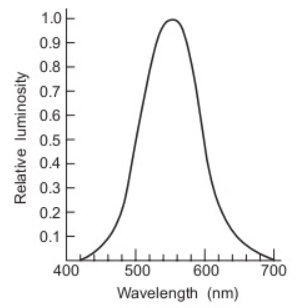Solution:

From the graph,

(a) The relative luminosity of wavelength 480 nm = 0.14.

(b) The relative luminosity of wavelength 520 nm = 0.68.

(c) The relative luminosity of wavelength 580 nm = 0.92.

(d) The relative luminosity of wavelength 600 nm = 0.66.

Question 4: The relative luminosity of wavelength 600 nm is 06. Find the radiant flux of 600 nm needed to produce the same brightness sensation as produced by 120 W of radiant flux at 555 nm.

Solution:

Relative luminosity is the ratio of “luminous flex of source of given wavelength” to the “luminous flex of source of 555 nm of sample power”

Relative luminosity = 0.6 (given)

Let “P” be the radiant flux of the source.

So, Luminous flux = 685P

=> 0.6 = [luminous flex of source of P watt]/685 P

=> 685 P x 0.6 = 120 x 685

=> P = 200 W

Question 5: The luminous flux of a monochromatic source of 1 w is 450 lumen watt-1. Find the relative luminosity at the wavelength emitted.

Solution:

Relative luminosity is the ratio of “luminous flex of source of given wavelength” to the “luminous flex of source of 555 nm of sample power”

=> Relative luminosity = 450/685 = 66%

Question 6: A source emits light of wavelengths 555 nm and 600 nm. The radiant flux of the 555 nm part is 40 W and of the 600 nm part is 30 W. The relative luminosity at 600 nm is 06. Find

(b) the total luminous flux,

(c) the luminous efficiency.

Solution:

(a) the total radiant flux = radiant flux of 555 nm part of light + radiant flux of 600 nm part of light = 40 W + 30 W = 70 W

(b) Total luminous flux = luminous flux of 555 nm part of light + luminous flux of 600 nm part of light = 1 × 40 × 685 + 0.6 × 30 × 685= 39730 lumen

(c) the luminous efficiency.

We know, Luminous efficiency = [total luminous flux]/[total radiant flux]

= 39730/70 = 567.6 lumen/W

Question 7: A light source emits monochromatic light of wavelength 555 nm. The source consumes 100 W of electric power and emits 35 W of radiant flux. Calculate the overall luminous efficiency.

Solution:

We know, overall luminous efficiency = [total luminous flux]/[power input]

= [35×685]/100

= 239.75 lumen/W

Question 8: A source emits 31.4 W of a radiant flux distributed uniformly in all directions. The luminous efficiency is 60 lumen watt-1. What is the luminous intensity of the source?

Solution:

Luminous intensity = [luminous flex]/[solid angle] …(1)

From question, Radiant flux = 31.4, since the radiant flux is distributed uniformly in all directions, the solid angle will be Luminous efficiency = 60 lumen/W

luminous flux = luminous efficiency × radiant flux= 60 × 31.4 lumen

(1)=> Luminous intensity = [60×31.4]/4π

= 150 candela

Question 9: A point source emitting 628 lumen of luminous flux uniformly in all directions is placed at the origin. Calculated the illuminance on a small area placed at (10 m, 0, 0) in such a way that the normal to the area makes an angle of 37° with the X-axis.

Solution:

We know, Luminous intensity = [luminous flex]/[solid angle] …(1)

Given: Luminous flux = 628 lumen

Distance of point, r = 1 m

Angle made by the normal with x-axis = 37 degrees

Since the radiant flux is distributed uniformly in all directions, the solid angle will be 4π.

(1)=> Luminous intensity = 628/4π = 50 candela

So, Illuminance, E = I cos (θ/r2)

=> E = 50 x [cos 37o]/12

= 40 lux

Question 10: The illuminance of a small area changes from 900 lumen to 400 lumen m-2 when it is shifted along its normal by 10 cm. Assuming that it is illuminated by a point source placed on the normal, find the distance between the source and the area in the original position.

Solution:

Let I be Luminous intensity of source.

Let EA = Initial illuminance = 900 lm/m2 and

EB = Final illuminance = 400 lumen/m2

Now,

Illuminance on the initial position = EA = [I cosθ]/x2 …(1)

Illuminance at final position = EB = [I cosθ]/(x+10) 2 …(2)

Now, (1) = (2)

=> I = [EA x2]/cosθ = [EB (x+1) 2]/cosθ

=> 900 x2 = 400(x + 10) 2

=> x = 20 cm

The distance between the source and the area at the initial position is 20 cm.

Question 11 : A point source emitting light uniformly in all directions is placed 60 cm above a table-top. The illuminance at a point on the table-top, directly below the source, is 15 lux. Find the illuminance at a point on the table-top 80 cm away from the first point.

Solution: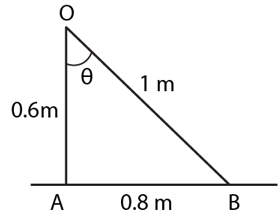EA = 15 lux = Io/602

=> Io = 15 x (0.6) 2 = 5.4 candela

And, EB = [Io cosθ] /(OB)2

= [5.4 x 3/5]/12

= 3.24 lux

Question 12: Light from a point source falls on a small area placed perpendicular to the incident light. If the area is rotated about the incident light by an angle of 60°, by what fraction will the illuminance change?

Solution:

The illuminance will not change.

Question 13: A student is studying a book placed near the edge of a circular table of radius R. A point source of light is suspended directly above the center of the table. What should be the height of the source above the table so as to produce maximum illuminance at the position of the book?

Solution:

Let the height of the source be h and the luminous intensity in the normal direction be Io.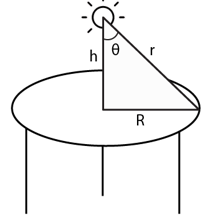The illuminance on book, E = [Io cosθ] /(r)2

From diagram, cosθ = h/r

=>E = [Io h] /(r)3

But r = √[R2+h2]

=> E = [Io h] /(R2+h2)3/2

For maximum illuminance, dE/dh = 0

Now,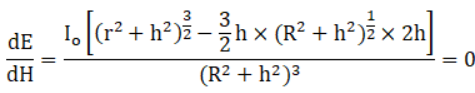=>R2 – 2h2 = 0

Or h = R/√2

Question 14: Figure (below) shows a small diffused plane source S placed over a horizontal table-top at a distance of 24 m with its plane parallel to the table-top. The illuminance at the point A directly below the source is 25 lux. Find the illuminance at a point B of the table at a distance of 18 m from A.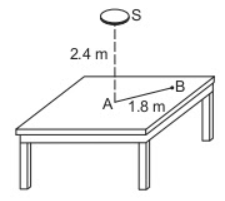Solution:

Given: Illuminance at A = 25 lux.

Form figure, AS = 2.4 m and AB = 1.8 m.

BS = √[(2.4) 2 + (1.8) 2] = 3 m

Let angle ASB is θ

=> cos θ = 2.4/3 = 0.8

We know, E = I cosθ/r2

=> 25 = Io cos0o/(2.4)2

Where Io is the intensity along SA

=> Io = 25 x 5.76 = 144 cd

Now, from Lambert’s cosine law, the intensity along SB:

I = Io cosθ

= 144 x 0.8

= 115.2 cd

The angle between SB and normal at B is also θ.

Illuminance at B is E = (I cosθ)/32 = 10.24 Lux

Question 15: An electric lamp and a candle produce equal illuminance at a photometer screen when they are placed at 80 cm and 20 cm from the screen respectively. The lamp is now covered with a thin paper which transmits 49% of the luminous flux. By what distance should be lamp be moved to balance the intensities at the screen again?

Solution:

I1/I2 = (80/20)2 = 16

Here I1 = Intensity when placed at a distance 80 cm and

I2 = Intensity when placed at a distance 20 cm apart from the screen.

Let the new distance between the lamp and the screen be x

0.49I1/I2 = (x/20)2

=> 0.49 x 16 x 400 = x2

=> x = 56 cm

The lamp has to be moved by 80 cm – 56 cm = 24 cm.

Question 16: Two light sources of intensities 8 Cd and 12 Cd placed on the same side of a photometer screen at a distance of 40 cm from it. Where should an 80 Cd source be placed to balance the illuminance?

Solution:

Total intensity of the 8 Cd and the 12 Cd light source is (8+12) = 20 Cd.

Illuminance due to the 20 Cd source E1 is:

E1 = 20/(0.4)2 …..(1)

Illuminance due to the 80 Cd source E2 is:

E2 = 80/(d)2 …..(1)

where d is the distance of the 80 Cd source.

Now,

E1 = E2

20/(0.4)2 = 80/(d)2

=> d = 0.8 m = 80 cm